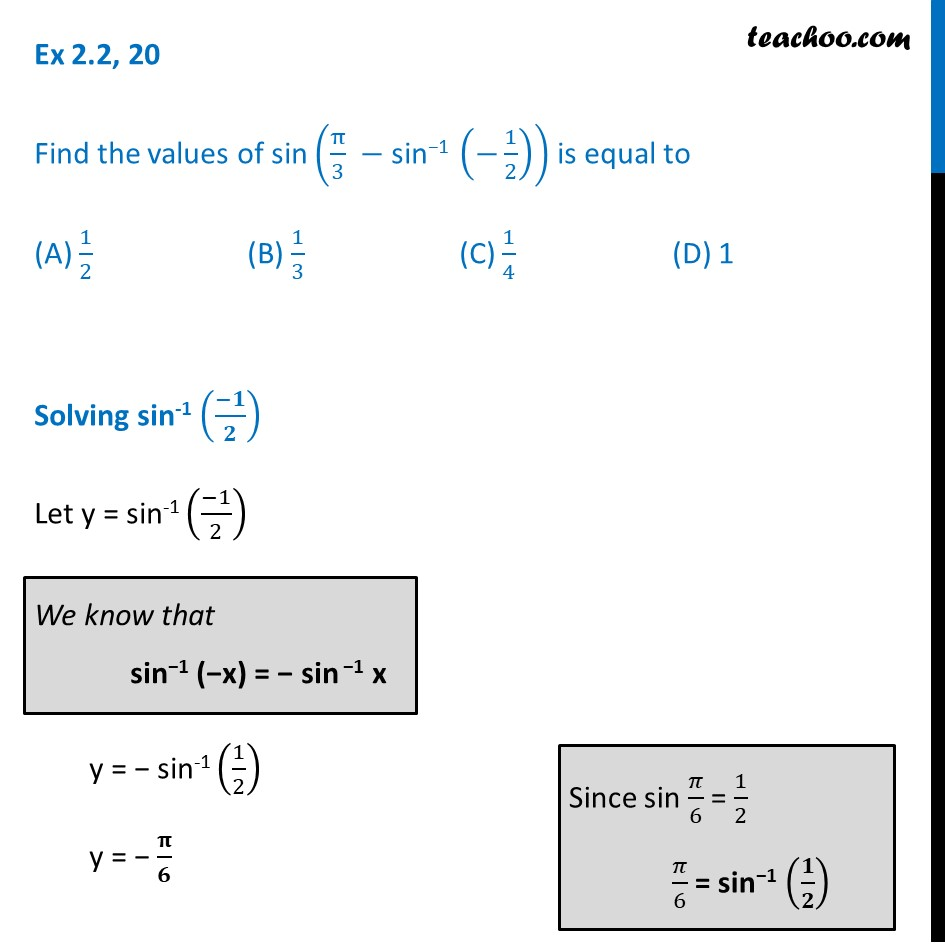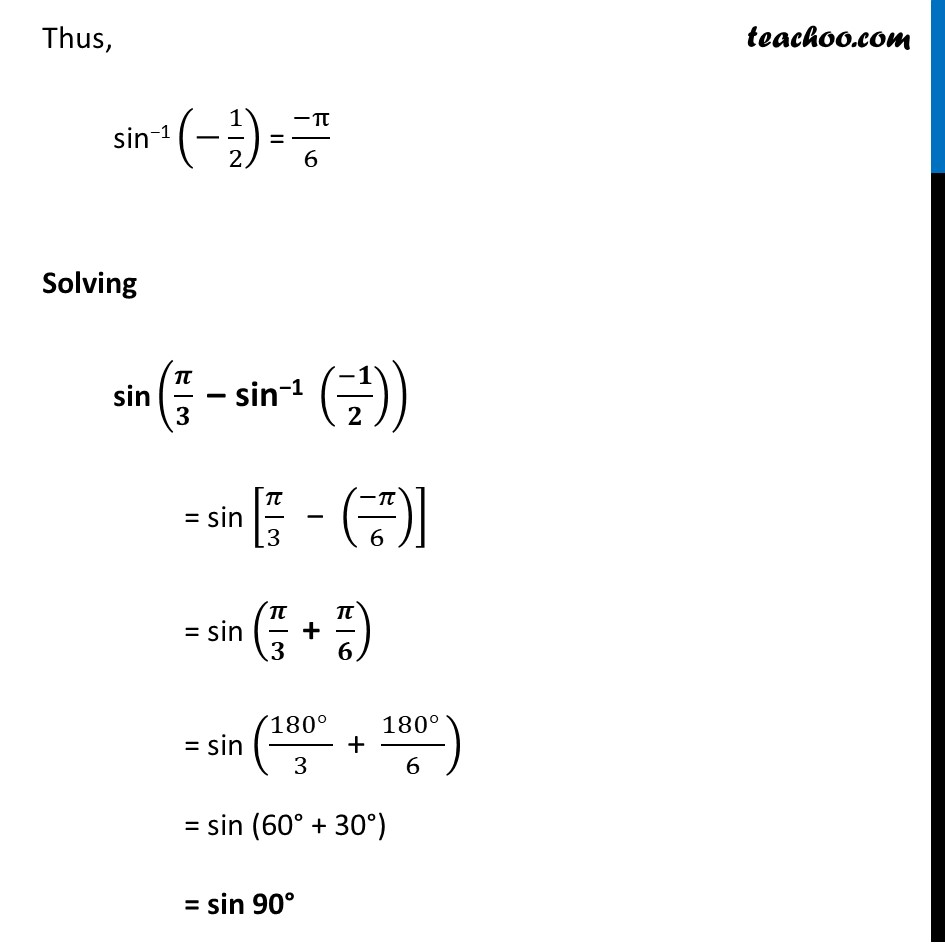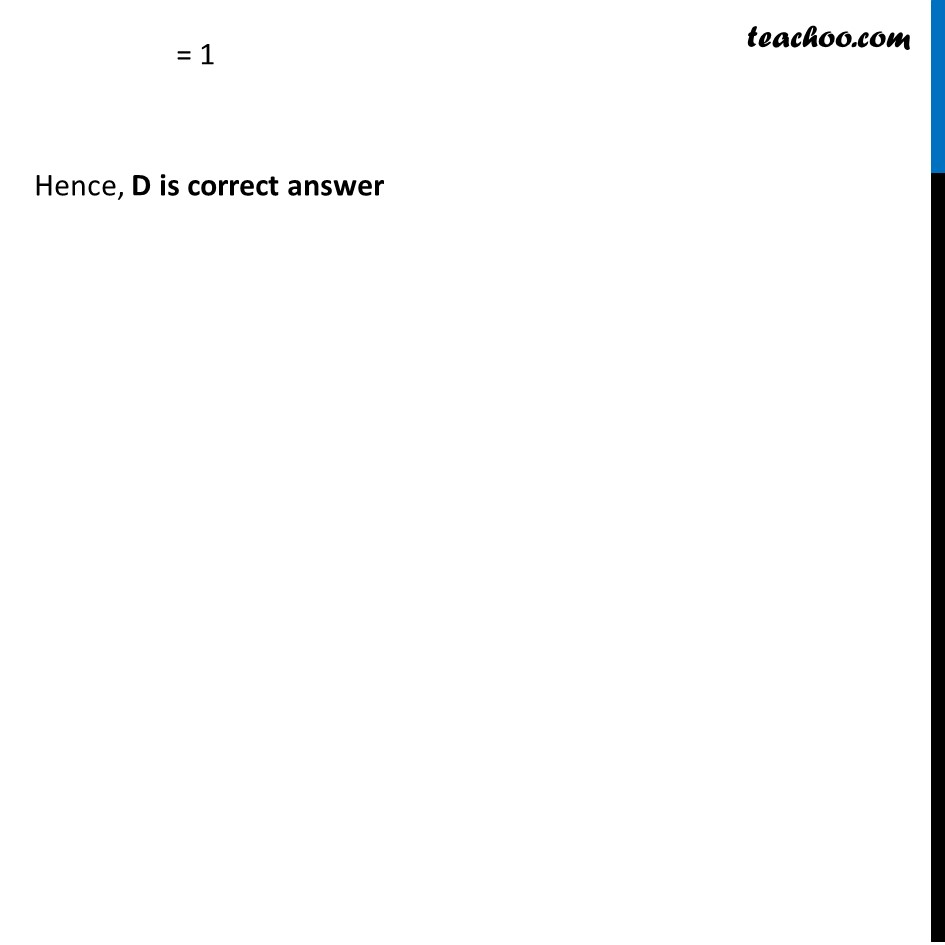Finding principal value

Chapter 2 Class 12 Inverse Trigonometric Functions
Concept wiseLearn in your speed, with individual attention - Teachoo Maths 1-on-1 Class

### Transcript

Ex 2.2, 20 Find the values of sin (π/3 −"sin−1" (−1/2)) is equal to (A) 1/2 (B) 1/3 (C) 1/4 (D) 1 Solving sin-1 ((−𝟏)/𝟐) Let y = sin-1 ((−1)/2) y = − sin-1 (1/2) y = − 𝛑/𝟔 We know that sin−1 (−x) = − sin −1 x Since sin 𝜋/6 = 1/2 𝜋/6 = sin−1 (𝟏/𝟐) Thus, sin−1 (−1/2) = (−π)/6 Solving sin (𝝅/𝟑 " – sin−1 " ((−𝟏)/𝟐)) = sin [𝜋/3 " − " ((−𝜋)/6)] = sin (𝝅/𝟑 " + " 𝝅/𝟔) = sin ((180° )/3 " + " (180° )/6) = sin (60° + 30°) = sin 90° = 1 Hence, D is correct answer